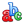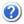Guest |Rus ||Просмотр примитивных и составных графиковПросмотр примитивных и составных графиков спектральных и иных функций (молекул и слабосвязанных молекулярных комплексов)Составной график
 Рисунок 106. Experimental Н2О self-continuum (T =296°K) versus predicted absorption spectra of bound and metastable dimers1 and present calculations for the 3500-3900 cm-1 band. The black dots are the experimental data, the triangles are the stable dimer absorption coefficients, the black curves are the quasi-bound dimer absorption coefficients, and the gray curves represent the calculated results using the κLorχ line shape. The frequency step is 50 cm-1.
Библиографическая ссылка Составной график
Olga B. Rodimova, Continuum water vapor absorption in the 4000–8000 cm-1 region, Proc. SPIE 9680, 21st International Symposium Atmospheric and Ocean Optics: Atmospheric Physics, SPIE - The international society for optical engineering, 2015, Pages 968002, DOI: 10.1117/12.2205332.
Координатная система (Линейный, Линейный)
Ось абсцисс (X) (Размерность) Волновое число (см⁻¹) Ось ординат (Y) (Размерность) Коэффициент поглощения (см²мол⁻¹атм⁻¹)
Рисунок 106. Original calculated results. Experimental Н2О self-continuum (T =296°K) versus predicted absorption spectra of bound and metastable dimers  and present calculations for the 3500-3900 cm-1 band. The gray curves represent the calculated results using the κLorχ line shape. The frequency step is 50 cm-1.Вещество H2O Тип данных Теоретические
Температура 296 К Метод измерения (решения), Модель, Аппроксимация
Давление (полное) Происхождение данных Оцифрованные
Давление (парциальное) Тип источника Первичный
Длина пути (L)
Плотность (d)
Волновое число (T зависимость)

Рисунок 106. Ptashnik, et al. (2011). Experimental Н2О self-continuum (T =296°K) versus predicted absorption spectra of bound and metastable dimers  and present calculations for the 3500-3900 cm-1 band.
 Ptashnik, I.V., Shine, K.P. and Vigasin, A.A., “Water vapour self-continuum and water dimers: 1. Analysis of recent work,” J. Quant. Spectrosc. and Radiat. Transfer. 112, 1286-1303 (2011).Вещество H2O Тип данных Экспериментальные
Температура 296 К Метод измерения (решения), Модель, Аппроксимация
Давление (полное) Происхождение данных Оцифрованные
Давление (парциальное) Тип источника Цитируемый
Длина пути (L)
I.V. Ptashnik, K.P. Shine, A.A. Vigasin, Water vapour self-continuum and water dimers: 1. Analysis of recent work, Journal of Quantitative Spectroscopy and Radiative Transfer, 2011, Volume 112, Issue 8, Pages 1286–1303, DOI: 10.1016/j.jqsrt.2011.01.012.
Плотность (d)
Волновое число (T зависимость)

Рисунок 106. Quasi-bound dimer absorption coefficients. Experimental Н2О self-continuum (T =296°K) versus predicted absorption spectra of bound and metastable dimers  and present calculations for the 3500-3900 cm-1 band. The  black curves are the quasi-bound dimer absorption coefficients. The frequency step is 50 cm-1.Вещество H2O-H2O Тип данных Теоретические
Температура 296 К Метод измерения (решения), Модель, Аппроксимация
Давление (полное) Происхождение данных Оцифрованные
Давление (парциальное) Тип источника Цитируемый
Длина пути (L)
Плотность (d)
Волновое число (T зависимость)

Рисунок 106. Stable dimer absorption coefficients. Experimental Н2О self-continuum (T =296°K) versus predicted absorption spectra of bound and metastable dimers  and present calculations for the 3500-3900 cm-1 band. The triangles are the stable dimer absorption coefficients.Вещество H2O-H2O Тип данных Теоретические
Температура 296 К Метод измерения (решения), Модель, Аппроксимация
Давление (полное) Происхождение данных Оцифрованные
Давление (парциальное) Тип источника Цитируемый
Длина пути (L)
Плотность (d)
Волновое число (T зависимость)
Поиск примитивного или составного графика в списке статей